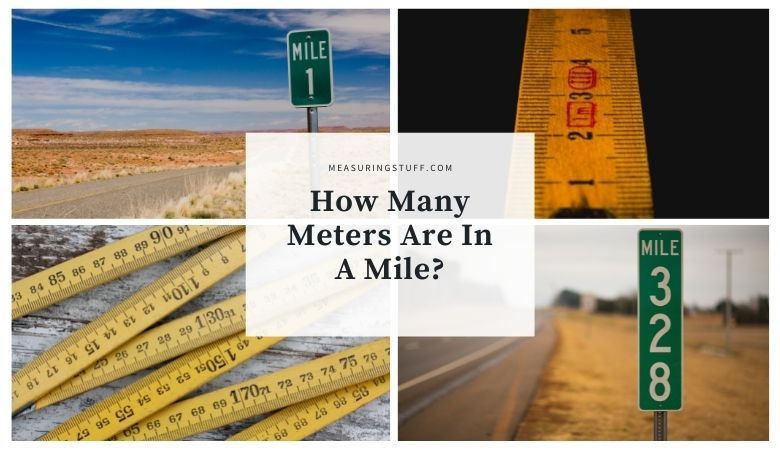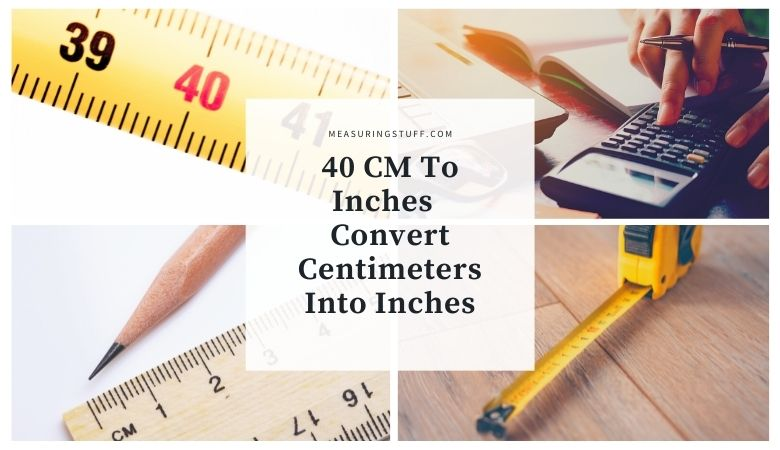# Length/Distance## How Many Meters Are In A Mile?

Although they’re parts of different measurement systems, meters and miles are both used to measure distance. Meters are a shorter measurement and are almost the same length as a yard, or 3 feet. Miles, on the other hand, are a much longer measurement, and they are often used to measure distance traveled by driving. There …## How Many Millimeters Are In A Centimeter?

Millimeters and centimeters are a unit of length measurement. Converting between the two units is quite simple.  There are 10 millimeters in 1 centimeter. To find out how many millimeters there are in any given number of centimeters, you can multiply the number of centimeters by 10. For example, if you want to find out …## 40 CM To Inches – Convert Centimeters Into Inches

Converting centimeters into inches can be done easily by using a formula, using a conversion calculator, or using a quick reference chart. I will show you all 3 methods. If you wish to convert 40 centimeters into inches, simply divide the number by 2.54. For example, 40cm / 2.54 = 15.748 inches. Regardless of how …